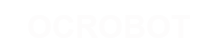#### Site Tools

en:reference:language:arithmetic

# Differences

This shows you the differences between two versions of the page.

 — en:reference:language:arithmetic [2017/04/07 10:03] (current) 2014/11/13 14:27 弘毅 created 2014/11/13 14:27 弘毅 created Line 1: Line 1: + ====== Addition, Subtraction,​ Multiplication,​ & Division ====== + + + ===== Description ===== + + These operators return the sum, difference, product, or quotient (respectively) of the two operands. The operation is conducted using the data type of the operands, so, for example, 9 / 4 gives 2 since 9 and 4 are ints. This also means that the operation can overflow if the result is larger than that which can be stored in the data type (e.g. adding 1 to an int with the value 32,767 gives -32,768). If the operands are of different types, the "​larger"​ type is used for the calculation. + + + If one of the numbers (operands) are of the type float or of type double, floating point math will be used for the calculation. + + + ===== Examples ===== + + y = y + 3; + x = x - 7; + i = j * 6; + r = r / 5; + ​ + ===== Syntax ===== + + result = value1 + value2; + result = value1 - value2; + result = value1 * value2; + result = value1 / value2; + ​ + ===== Parameters: ===== + + value1: any variable or constant + value2: any variable or constant + + + ===== Programming Tips: ===== + + * Know that integer constants default to int, so some constant calculations may overflow (e.g. 60 * 1000 will yield a negative result). + + + * Choose variable sizes that are large enough to hold the largest results from your calculations + + + * Know at what point your variable will "roll over" and also what happens in the other direction e.g. (0 - 1) OR (0 - - 32768) + + + * For math that requires fractions, use float variables, but be aware of their drawbacks: large size, slow computation speeds + + + * Use the cast operator e.g. (int)myFloat to convert one variable type to another on the fly. +